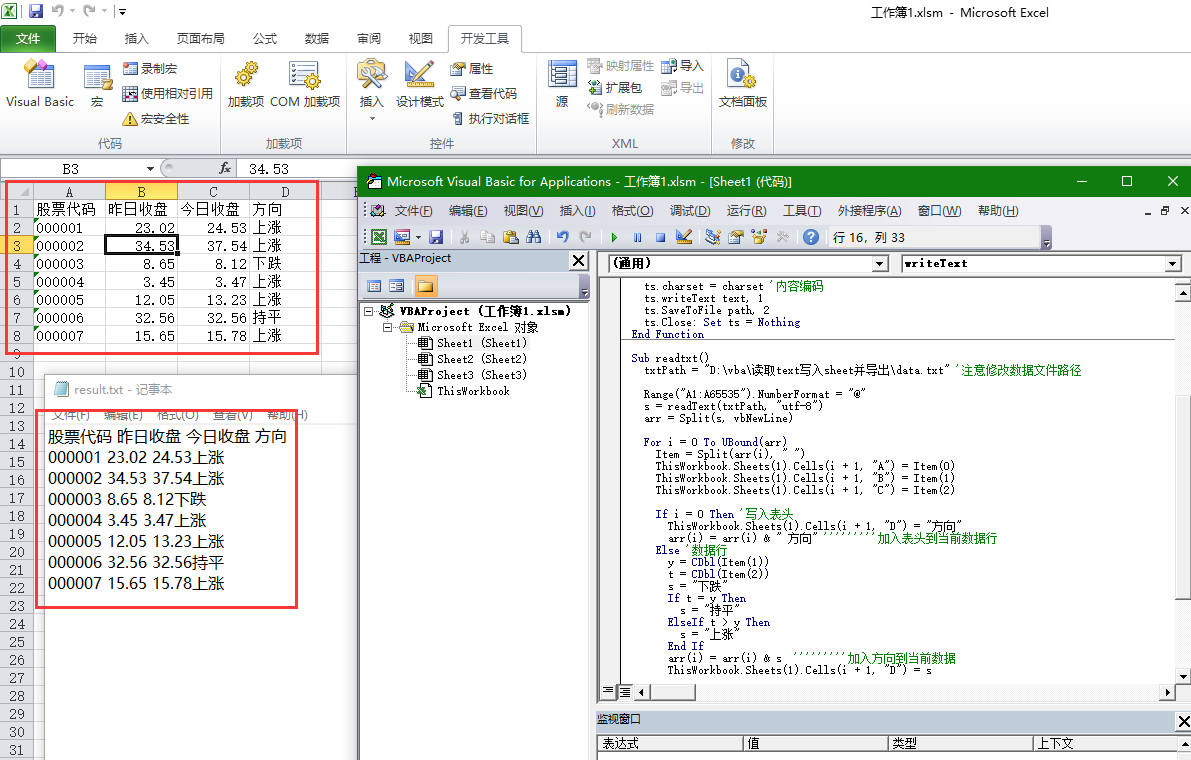2021-07-06 15:14

# 编写VBA程序，从文本文档中读取数据到Excel表格中，在复制入另一个文本文档中

000001 23.02 24.53
000002 34.53 37.54
000003 8.65 8.12
000004 3.45 3.47
000005 12.05 13.23
000006 32.56 32.56
000007 15.65 15.78

• 点赞
• 写回答
• 关注问题
• 收藏
• 邀请回答

#### 2条回答默认 最新

•CSDN专家-showbo 2021-07-06 15:27
已采纳

用Scripting.FileSystemObject对象读取text文件后split下得到行在遍历行写入当前运行vba的xlsm第一个工作簿中就行了

点赞 打赏 评论
•``````Function readText(path, charset) '读文件
ts.charset = charset '内容编码
ts.Mode = 3 '读写模式
ts.Type = 2 '文本模式
ts.Open
ts.Close: Set ts = Nothing
End Function
Function writeText(path, charset, text) '写文件
ts.Mode = 3 '写写模式
ts.Type = 2 '文本模式
ts.Open
ts.charset = charset '内容编码
ts.writeText text, 1
ts.SaveToFile path, 2
ts.Close: Set ts = Nothing
End Function

txtPath = "D:\vba\读取text写入sheet并导出\data.txt" '注意修改数据文件路径

Range("A1:A65535").NumberFormat = "@"
arr = Split(s, vbNewLine)

For i = 0 To UBound(arr)
Item = Split(arr(i), " ")
ThisWorkbook.Sheets(1).Cells(i + 1, "A") = Item(0)
ThisWorkbook.Sheets(1).Cells(i + 1, "B") = Item(1)
ThisWorkbook.Sheets(1).Cells(i + 1, "C") = Item(2)

If i = 0 Then '写入表头
ThisWorkbook.Sheets(1).Cells(i + 1, "D") = "方向"
arr(i) = arr(i) & " 方向" '''''''''加入表头到当前数据行
Else '数据行
y = CDbl(Item(1))
t = CDbl(Item(2))
s = "下跌"
If t = y Then
s = "持平"
ElseIf t > y Then
s = "上涨"
End If
arr(i) = arr(i) & s  '''''''''加入方向到当前数据
ThisWorkbook.Sheets(1).Cells(i + 1, "D") = s
End If
Next

'join下写回新文件中
s = Join(arr, vbNewLine)
newtxtPath = "D:\vba\读取text写入sheet并导出\result.txt" '注意修改结果路径

writeText newtxtPath, "utf-8", s '写回文件

End Sub

``````
点赞 打赏 评论